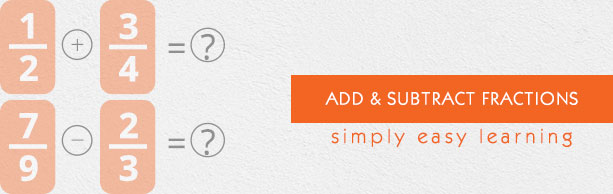# Add and Subtract Fractions

This tutorial provides comprehensive coverage of adding and subtracting of fractions based on Common Core (CCSS) and State Standards and its prerequisites. Students can navigate learning paths based on their level of readiness. Institutional users may customize the scope and sequence to meet curricular needs. This simple tutorial uses appropriate examples to help you understand adding and subtracting of fractions in a general and quick way.

# Audience

This tutorial has been prepared for beginners to help them understand the basics of adding and subtracting of fractions. After completing this tutorial, you will find yourself at a moderate level of expertise in adding and subtracting of fractions, from where you can advance further.

# Prerequisites

Before proceeding with this tutorial, you need a basic knowledge of elementary math concepts such as number sense, addition, subtraction, multiplication, division, whole numbers, fractions, equivalent fractions, least common denominator and so on.